Question

A circles has radius 10 centimeters. Suppose an arc on the circle has length 8\pi centimeters. what is the measure of the central angle whose radii define the arc?

1.RuslanHeatt

The measure of the central angle whose radii define the arc is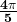Step-by-step explanation:

Radius of circle = 10 cm

Length of arc =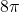We need to find Theta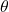The formula used will be: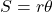S= length of arc, r = radius and= angle

Putting values and finding \theta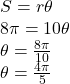So, the measure of the central angle whose radii define the arc is